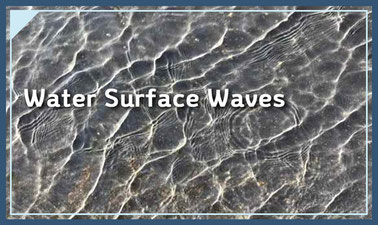# Water Surface Waves: Finite-amplitude waves

To understand non-linear water wave theories and perturbation methodsThis course is archived
Future dates to be announced
Estimated 4 weeks
4–6 hours per week
Self-paced
Free

This course covers fundamentals of nonlinear water waves based on perturbation methods. After reviewing basic techniques of perturbation methods, we study solutions for Stokes waves of deep water. After identifying the limitation of Stokes waves solution in shallow water, we then derive the nonlinear shallow-water equations. Finally, we explore the Boussinesq equations to account for (weak) dispersion and solve the kdV equation to obtain the solitary wave solution.

### At a glance

• Institution: SNUx
• Subject: Engineering
• Prerequisites:

It is recommended to take a previous lecture, Water Surface Waves: Small Amplitude Waves, before this lecture.

• Language: English

# What you'll learn

Skip What you'll learn

l Perturbation methods

l Stokes wave theory and solutions

l Nonlinear shallow water equations

l Boussinesq equations

l kdV equation and solitary wave solution

# Syllabus

Skip Syllabus

Week 1. Introduction to perturbation method

Week 2. Stokes wave theory

Week 3. Nonlinear shallow-water equations

Week 4. Boussinesq equations and KdV equation